Name:     ID:

Email:

MDM4U - Unit 6 Quiz

True/False
Indicate whether the statement is true or false.

1.

If the correlation coefficient is negative, the relationship slopes downward to the right.

2.

A data set with r = 0.9 has a stronger fit than a data set with r = –0.9.

3.

An outlier can reduce the accuracy of a linear model.

Multiple Choice
Identify the choice that best completes the statement or answers the question.

4.

Which value of r would be appropriate for the scatter plot shown?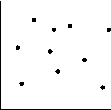a. 0.4 c. –0.4 b. 0 d. 0.9

5.

Which value of r would be appropriate for the scatter plot shown?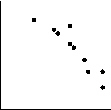a. –0.9 c. –0.5 b. 0.9 d. 0.5

6.

The scatter plot shown includes an outlier in the upper left corner of the graph. The line of best fit is shown.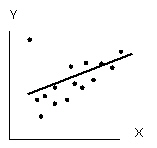How would the line of best fit be affected if the outlier were removed?
 a. The slope would decrease and remain positive. b. The slope would decrease and become negative. c. The slope would increase. d. The slope would be unchanged.

7.

Which error in the use of linear regression might be present?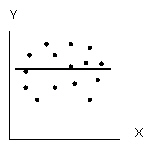a. too few data points b. an outlier was not removed from the data set c. the model is not linear d. all of the above

8.

Which error in the use of linear regression might be present?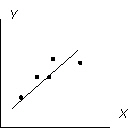a. too few data points b. an outlier was not removed from the data set c. the model is not linear d. all of the above

9.

Using a linear-regression equation to predict values between actual data points is an example of
 a. extrapolation c. least-squares fit b. residuals d. interpolation

10.

For the line of best fit in the least-squares method,
 a. the sum of the squares of the residuals has the greatest possible value b. the sum of the squares of the residuals has the least possible value c. the sum of the residuals is equal to one d. both b) and c)

A university conducted a study to compare first year class sizes with average marks. Ten classes were selected at random. The results are shown in the table.
 ClassSize, c 123 102 88 95 147 75 43 110 98 176 MeanMark, m 64.9 69.4 72.2 58.0 62.1 73.0 74.5 66.3 69.8 60.1

The line of best fit has equation m = –0.114c + 79.085 with r = –0.743

11.

Classify the linear correlation.
 a. strong, positive c. moderate, positive b. strong, negative d. moderate, negative

12.

Which data point might be considered an outlier?
 a. (102, 69.4) c. (176, 60.1) b. (75, 73.0) d. (95, 58.0)

13.

For a class size of 147 students, the model predicts a mean mark of
 a. 62.3 c. 62.1 b. 64.9 d. 70.4